SLVS947C October   2009  – August 2014

PRODUCTION DATA.

1. Features
2. Applications
3. Description
4. Simplified Schematics
5. Revision History
6. Pin Configuration and Functions
7. Specifications
8. Detailed Description
1. 8.1 Overview
2. 8.2 Functional Block Diagrams
3. 8.3 Feature Description
4. 8.4 Device Functional Modes
9. Application and Implementation
1. 9.1 Application Information
2. 9.2 Typical Application
3. 9.3 Typical Application Circuit, TSSOP
10. 10Power Supply Recommendations
11. 11Layout
12. 12Device and Documentation Support
13. 13Mechanical, Packaging, and Orderable Information

• PW|24
• RGE|24
• RGE|24

## 9 Application and Implementation

### 9.2 Typical Application

The TPS53125 is a Dual D-CAP2™ Mode Control Step-Down Controller in a realistic cost-sensitive application. Providing both a low core-type 1.05 V and I/O type 1.8 V output from a loosely regulated 12 V source. Idea applications are: Digital TV Power Supply, Networking Home Pin, Digital Set-Top Box (STB), DVD Player/Recorder, and Gaming Consoles.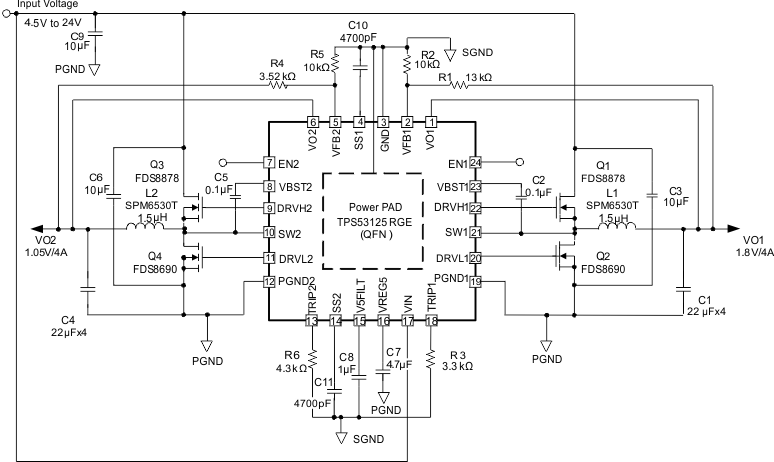Figure 6. TPS53125 Typical Application Circuit (QFN)

### Table 1. Design Parameters

PARAMETERS EXAMPLE VALUES
Input voltage 12 V
Output voltage VO1 = 1.8 V, VO2 = 1.05 V

#### 9.2.2.1 Choose Inductor

The inductance value is selected to provide approximately 30% peak to peak ripple current at maximum load. Larger ripple current increases output ripple voltage, improve S/N ratio and contribute to stable operation.

Equation 3 can be used to calculate L1.

Equation 3.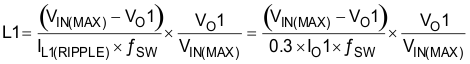The inductors current ratings needs to support both the RMS (thermal) current and the Peak (saturation) current. The RMS and peak inductor current can be estimated as follows.

Equation 4.Equation 5.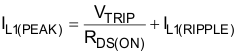Equation 6.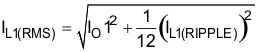Note: The calculation above shall serve as a general reference. To further improve transient response, the output inductance could be reduced further. This needs to be considered along with the selection of the output capacitor.

#### 9.2.2.2 Choose Output Capacitor

The capacitor value and ESR determines the amount of output voltage ripple and load transient response. it is recommended to use a ceramic output capacitor.

Equation 7.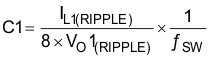Equation 8.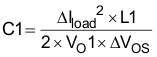Equation 9.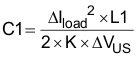Where

Equation 10.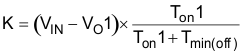Select the capacitance value greater than the largest value calculated from Equation 7, Equation 8 and Equation 9. The capacitance for C1 should be greater than 66 μF.

Where

ΔVOS = The allowable amount of overshoot voltage in load transition

ΔVUS = The allowable amount of undershoot voltage in load transition

Tmin(off) = Minimum off time

#### 9.2.2.3 Choose Input Capacitor

The TPS53125 requires an input decoupling capacitor and a bulk capacitor is needed depending on the application. A minimum 10-μF high-quality ceramic capacitor is recommended for the input capacitor. The capacitor voltage rating needs to be greater than the maximum input voltage.

#### 9.2.2.4 Choose Bootstrap Capacitor

The TPS53125 requires a bootstrap capacitor from SW to VBST to provide the floating supply for the high-side drivers. A minimum 0.1-μF high-quality ceramic capacitor is recommended. The voltage rating should be greater than 10 V.

#### 9.2.2.5 Choose VREG5 and V5FILT Capacitor

The TPS53125 requires both the VREG5 regulator and V5FILT input are bypassed. A minimum 4.7-μF high-quality ceramic capacitor must be connected between the VREG5 and GND for proper operation. A minimum 1-μF high-quality ceramic capacitor must be connected between the V5FILT and GND for proper operation. Both of these capacitors’ voltage ratings should be greater than 10 V.

#### 9.2.2.6 Choose Output Voltage Set Point Resistors

The output voltage is set with a resistor divider from the output voltage node to the VFBx pin. It is recommended to use 1% tolerance or better resisters. Select R2 between 10 kΩ and 100 kΩ and use Equation 11 or Equation 12 to calculate R1.

Equation 11.Equation 12.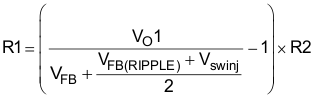Where

VFB(RIPPLE) = Ripple voltage at VFB

Vswinj = Ripple voltage at error comparator

#### 9.2.2.7 Choose Over Current Set Point Resistor

Equation 13.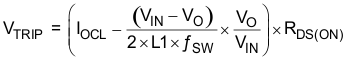Equation 14.Where

RDS(ON) = Low side FET on-resistance

ITRIP(min) = TRIP pin source current （8.5 μA）

VOCL0ff = Minimum over current limit offset voltage (–20 mV)

IOCL = Over current limit

#### 9.2.2.8 Choose Soft Start Capacitor

Soft start time equation is as follows.

Equation 15.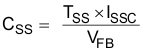#### 9.2.3 Application Curves (QFN)IO1 = 3 A VO1 = 1.8 V
Figure 7. Switching Frequency
vs Input Voltage (CH1)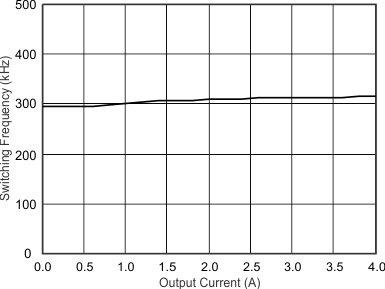VIN = 12 V VO1 = 1.8 V
Figure 9. Switching Frequency
vs Output Current (CH1)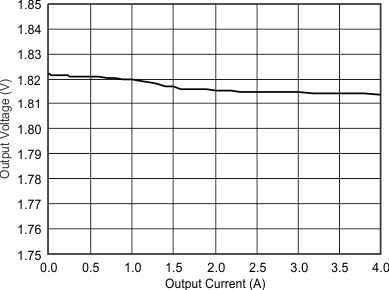VIN = 12 V VO1 = 1.8 V
Figure 11. Output Voltage
vs Output Current (CH1)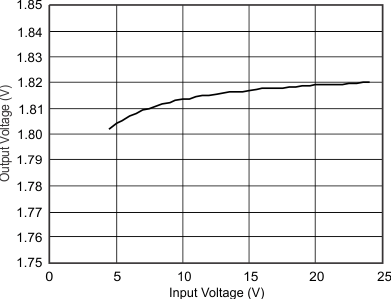VIN = 12 V VO1 = 1.85 V
Figure 13. Output Voltage
vs Input Voltage (CH1)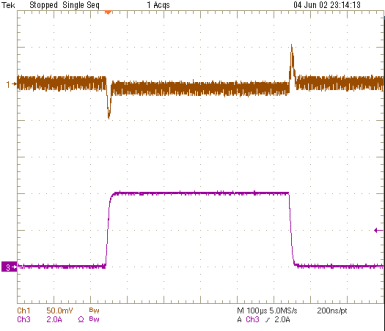Figure 17. Start-up Waveforms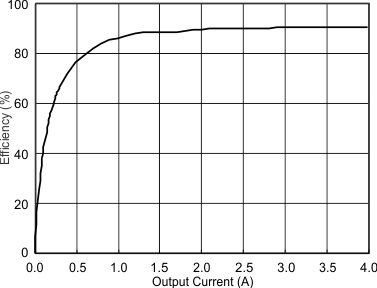VO1 = 1.8 V
Figure 19. 1.8-V Efficiency vs Output Current (CH1)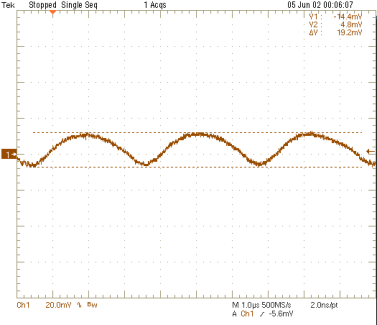VO1 = 1.8 V
Figure 21. 1.8-V Output Ripple Voltage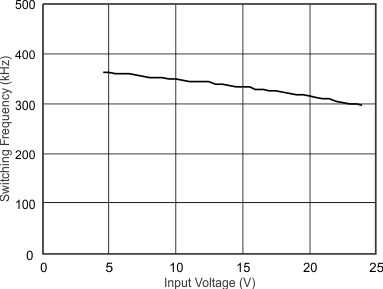IO2 = 3 A VO2 = 1.05 V
Figure 8. Switching Frequency
vs Input Voltage (CH2)VIN = 12 V VO2 = 1.05 V
Figure 10. Switching Frequency
vs Output Current (CH2)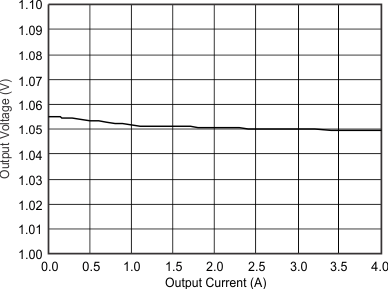VIN = 12 V VO2 = 1.05 V
Figure 12. Output Voltage
vs Output Current (CH2)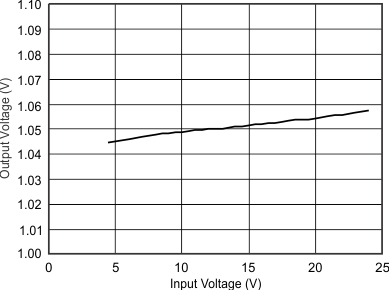VIN = 12 V VO2 = 1.05 V
Figure 14. Output Voltage
vs Input Voltage (CH2)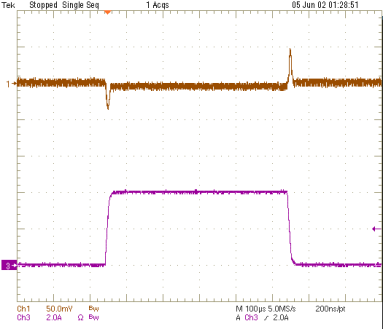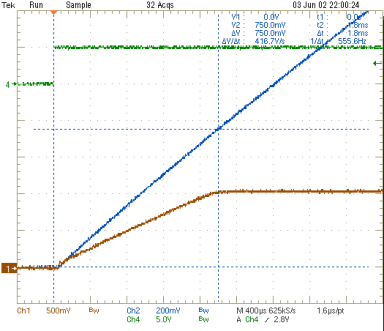Figure 18. Start-up Waveforms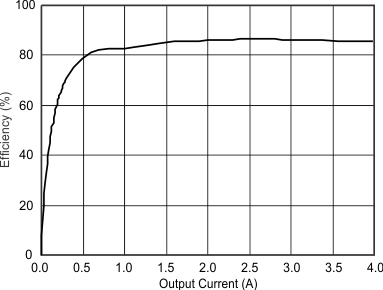VO2 = 1.05 V
Figure 20. 1.05-V Efficiency vs Output Current (CH2)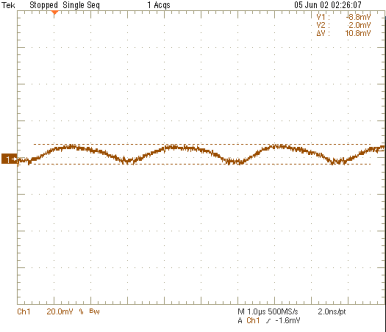VO2 = 1.05 V
Figure 22. 1.05-V Output Ripple Voltage

### 9.3 Typical Application Circuit, TSSOP

The TPS53125 is a Dual D-CAP2™ Mode Control Step-Down Controller in a realistic cost-sensitive application. Providing both a low core-type 1.05 V and I/O type 1.8V output from a loosely regulated 12 V source.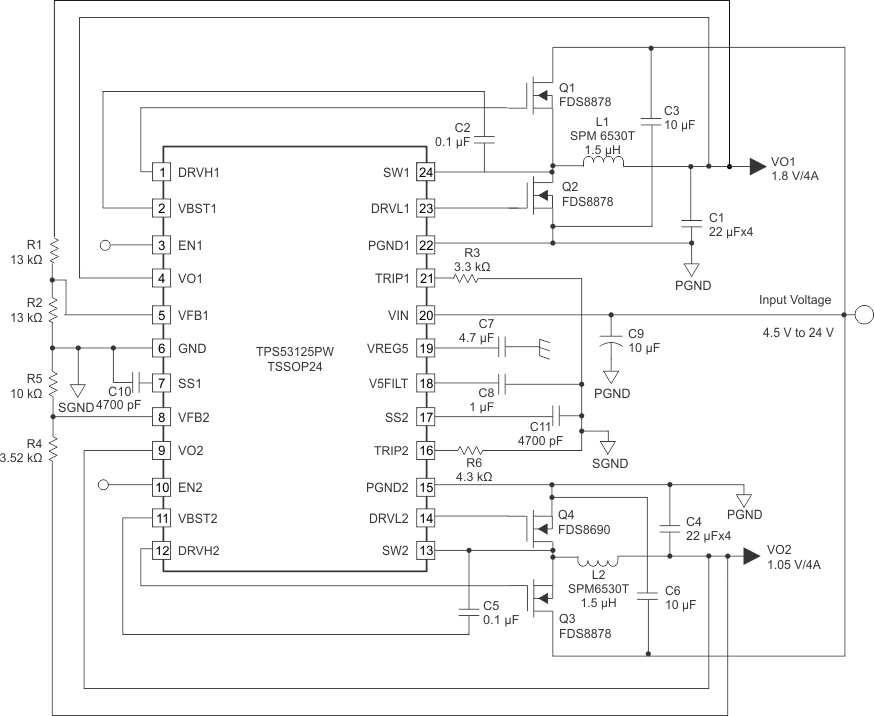Figure 23. TSSOP

#### 9.3.1 Design Requirements

For the Design Requirements, refer to Design Requirements (QFN).

#### 9.3.2 Detailed Design Procedure

For the Detailed Design Procedure, refer to Detailed Design Procedure (QFN).

#### 9.3.3 Application Curves

For Application Curves, refer to Application Curves (QFN).